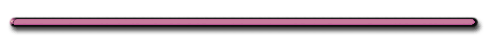HomeTotal Internal Reflection - PHET

showmethephysics Instruction

Total Internal Reflection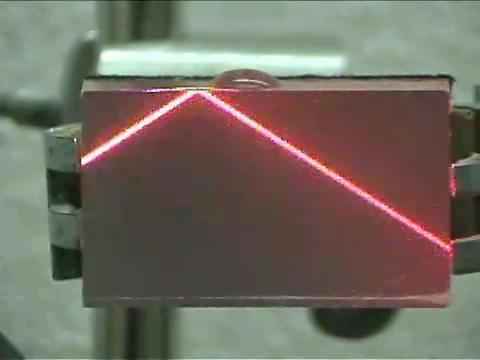| Rutgers University Physics |

Total Internal Reflection

internalReflection2_HR.wmv
St. Mary's University

[ Flash ]
Show Me The Physics

 Did you know ...   Skipping a rock across water has one thing in common with 'skipping' light off a media boundary.     Total Internal Reflection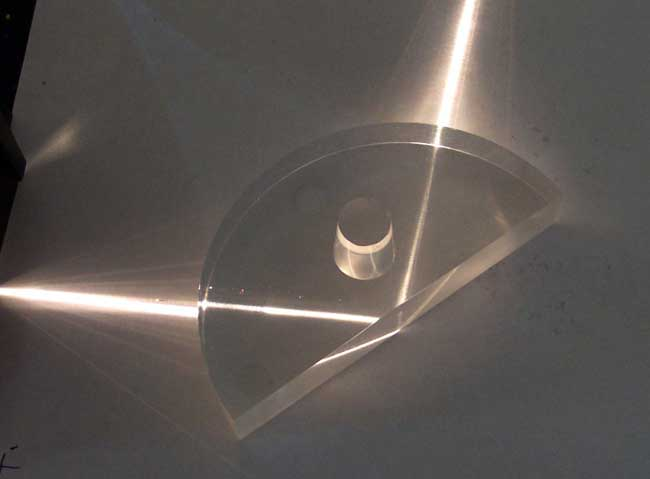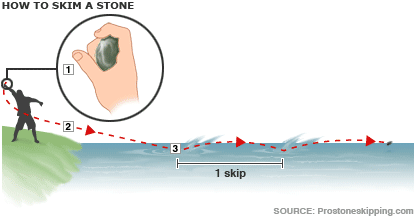A stone will skip across a pond only at wide angles from the normal (about 70 degrees)

2. Critical Angle θc– angle of incidence for which the angle of refraction equals 900 when light travels inside one medium that borders on air or a vacuum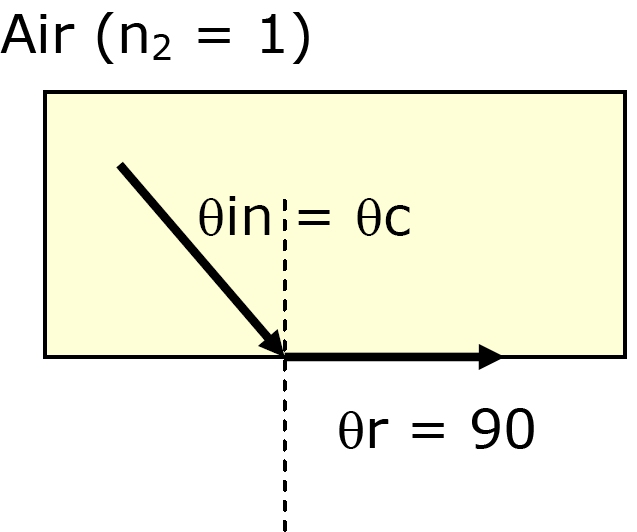Deriving Critical Angle Equation

n1sinθ1 = n2 sinθ2

Substitute θc, θ2 = 90, n2 = 1

n1sinθc = 1sin90

n1sinθc = 1

 sinθc = 1/n1

Ex) Find θc for Lucite

sin θc = 1/n1

From reference table, n1 = 1.50

sinθc = 1/1.50

θc = 41.8

3. Total Internal ReflectionTotal Internal Reflection [ HTML5 ] Show Me The Physics

What is this called?Rutgers University Physics

[ Flash ]

(These kinds of fibers are coated with materials that have a low index of refraction (fast).

When θ1 > θc
Total Internal Reflection

(Outside Medium Must Be Faster)

Trouble Viewing Video Above?

| Total Internal Reflection |

Rutgers U. Physics

Ex) Lucite

θc = 41.8Air

When θ1 = θc

θ2 = 900When θ < θc Refraction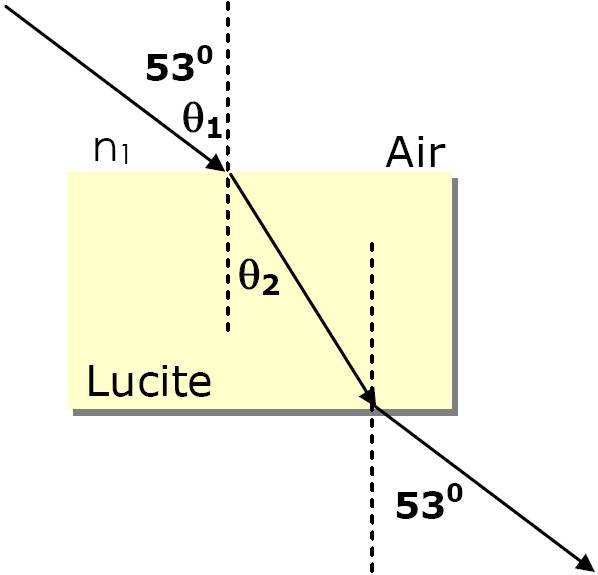Rick Reeda) Total Internal Reflection?Choice 2

(Outside Medium Must be Fast)

b) Refraction?Choice 3

c) Impossible?Choice 1

(Light always crosses over normal)

 What's wrong with this picture?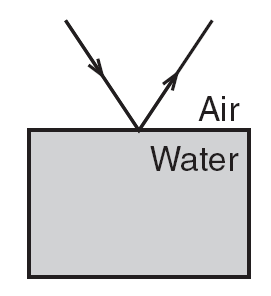Part of the ray would be refracted.   Total Internal Reflection only occurs when the outside  medium is very fast.DispersionEnrichment